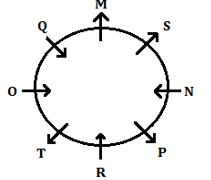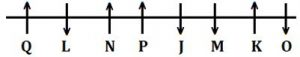Latest Banking jobs   »

# Reasoning Ability Quiz For IBPS Clerk/PO Prelims 2022- 5th September

Directions (1-5): In each of the questions below some statements are given followed by some conclusions. You have to take the given statements to be true even if they seem to be at variance with commonly known facts. Read all the conclusions and then decide which of the given conclusions logically follows from the given statements disregarding commonly known facts.
(a) If only conclusion I follows.
(b) If only conclusion II follows.
(c) If either conclusion I or II follows.
(d) If neither conclusion I nor II follows.
(e) If both conclusions I and II follow.

Q1. Statements: P > Y ≤ H ≥ U > T = N
Conclusions: I. P > U
II. N < H

Q2. Statements: A ≥ S > D = C ≥ V > F > G ≥ H
Conclusions: I. V < D
II. H ≤ V

Q3. Statements: F > G ≥ T ≥ H > Y, T ≤ L = J < K
Conclusions: I. J > H
II. J = H

Q4. Statements: B > N ≥ M = K > L, W ≤ E = B, Q ≥ W > A
Conclusions: I. W > M
II. Q ≤ K

Q5. Statements: C > V = B > N ≥ M, K ≤ L = O ≤ P < V
Conclusions: I. O < C
II. B > L

Directions (6-10): Study the following information carefully and answer the questions given below:
Eight persons M, N, O, P, Q, R, S and T are sitting around a circular table but not necessarily in the same order. Four of them are facing towards the center while rests are facing outside from the center of the table.
There are two persons sit between R and S and both are facing opposite direction to each other. P sits immediate to the right of R. Both O and Q are immediate neighbors to each other and facing in the same direction. Both P and S are not the immediate neighbors of both O and Q. Both O and N are facing to each other. T sits 3rd to the left of M, who does not sit opposite to S.

Q6. How many persons sit between Q and N, when counting from right of N?
(a) One
(b) None
(c) Two
(d) Three
(e) More than three

Q7. Who among the following sits opposite to M?
(a) O
(b) T
(c) P
(d) R
(e) N

Q8. Four of the following five are alike in a certain way and hence they form a group. Which one of the following does not belong to that group?
(a) Q, R
(b) O, N
(c) N, Q
(d) O, P
(e) R, S

Q9. What is the position of T with respect to P?
(a) 2nd to the right
(b) 2nd to the left
(c) Immediate to the right
(d) 3rd to the left
(e) 3rd to the right

Q10. If the number of persons sit between O and R, when counted to the left of O is same as the number of persons sit between __ and M, when counted to the left of ___?
(a) S
(b) Q
(c) R
(d) P
(e) N

Directions (11-15): Study the information carefully and answer the questions given below.
Eight persons J, K, L, M, N, O, P and Q are sitting in a linear row some of them facing towards the north and some of them facing towards south but not necessary in the same order. Not more than two consecutive persons face same direction.
N sits second to the right of J. Q sits second to the left of N. Two persons sit between Q and P. More than three persons sit between L and O. The persons sit on extreme ends face opposite direction to each other. M sits second to the right of P. N is not immediate neighbor of M, who sits third from one of the extreme ends of row. O is not immediate neighbor of N and M. O face south direction. J sits immediate right of M. L face opposite direction to Q.

Q11. Who among the following person sits second to right of O?
(a)M
(b)P
(c)J
(d)K
(e)None of these

Q12. How many persons sits between N and K?
(a) One
(b) Two
(c) Four
(d) Three
(e)None of these

Q13. Who among the following person is immediate neighbor of P and J?
(a) L
(b) M
(c) K
(d) Q
(e) None of these

Q14. How many persons sits on the right of M?
(a) One
(b) More than Four
(c) Three
(d) Four
(e) None

Q15. Four of the following five are alike in certain way based from a group, find the one that does not belong to that group?
(a) Q
(b) N
(c) K
(d) J
(e) P

Solutions

S1. Ans. (b)
Sol. I. P > U (False)
II. N < H (True)

S2. Ans. (d)
Sol. I. V < D (False)
II. H ≤ V (False)

S3. Ans. (c)
Sol. I. J > H (False)
II. J = H (False)

S4. Ans. (d)
Sol. I. W > M (False)
II. Q ≤ K (False)

S5. Ans. (e)
Sol. I. O < C (True)
II. B > L (True)

Solutions (6-10):
Sol.S6. Ans. (c)
S7. Ans. (d)
S8. Ans. (b)
S9. Ans. (a)
S10. Ans. (e)

Solutions (11-15):
Sol.S11. Ans. (a)
S12. Ans. (d)
S13. Ans. (e)
S14. Ans. (b)
S15. Ans. (d)#### Congratulations!Download Hindu Review of October 2021: Free PDF